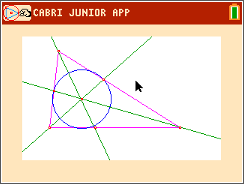### Special Segments in Triangles

Students will construct and explore medians, altitudes, angle bisectors, and perpendicular bisectors of triangles. They then drag the vertices to see where the intersections of the segments lie in relation to the triangle, and they measure distances to identify relationships.
•TI-84 Plus CE
•TI-84 Plus C Silver Edition
• TI-84 Plus Silver Edition
• TI-84 Plus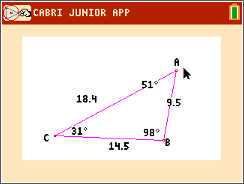### Triangle Sides & Angles

Students will explore side and angle relationships in a triangle. First, students will discover where the longest (and shortest) side is located relative to the largest (and smallest) angle. Then, students will explore the Isosceles Triangle Theorem and its converse. Finally, students will determine the number of acute, right, or obtuse angles that can exist in any one triangle.
•TI-84 Plus CE
•TI-84 Plus C Silver Edition
• TI-84 Plus Silver Edition
• TI-84 Plus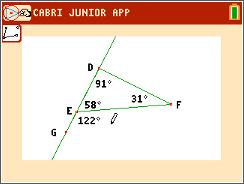### Exterior & Remote Interior Angles

Students investigate an exterior angle and its two remote interior angles.
•TI-84 Plus CE
•TI-84 Plus C Silver Edition
• TI-84 Plus Silver Edition
• TI-84 Plus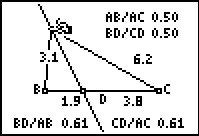### Angle Bisectors in a Triangle

Students explore the relationships between an angle bisector and segments in a triangle.
•TI-84 Plus CE
•TI-84 Plus C Silver Edition
• TI-84 Plus Silver Edition
• TI-84 Plus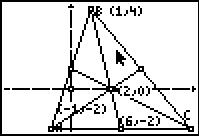### Balancing Point

In this activity, students will explore the median and the centroid of a triangle.
•TI-84 Plus CE
•TI-84 Plus C Silver Edition
• TI-84 Plus Silver Edition
• TI-84 Plus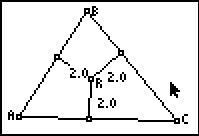### Hanging with the Incenter

In this activity, students will explore the perpendicular bisector theorem and discover that if a point is on the perpendicular bisector of a segment, then the point is equidistant from the endpoints.
•TI-84 Plus CE
•TI-84 Plus C Silver Edition
• TI-84 Plus Silver Edition
• TI-84 Plus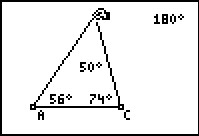### Interior & Exterior Angles of a Triangle

Students measure interior and exterior angles of a triangle and make conjectures about their relationships.
•TI-84 Plus CE
•TI-84 Plus C Silver Edition
• TI-84 Plus Silver Edition
• TI-84 Plus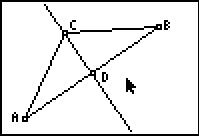### Perpendicular Bisector

Students will explore the perpendicular bisector theorem and discover that if a point is on the perpendicular bisector of a segment, then the point is equidistant from the endpoints.
•TI-84 Plus CE
•TI-84 Plus C Silver Edition
• TI-84 Plus Silver Edition
• TI-84 Plus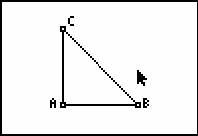### Special Triangles

Students visualize the construction of special right triangles and find the ratios of the sides.
•TI-84 Plus CE
•TI-84 Plus C Silver Edition
• TI-84 Plus Silver Edition
• TI-84 Plus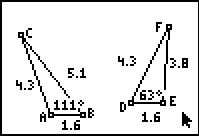••